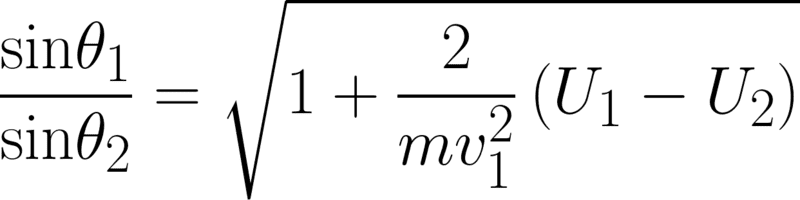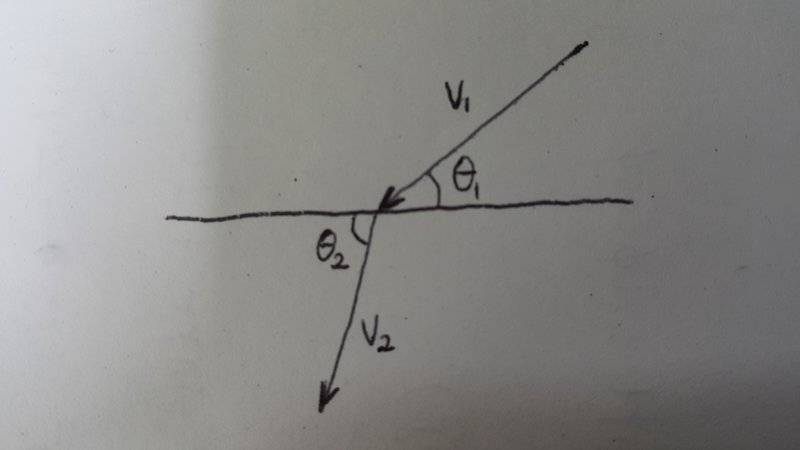# Landau Vol.1 Mechanics(3rd ed.) Ch.II §7. Momentum Problem

• jinjung

## Homework Statement

A particle of mass m moving with velocity v1 leaves a half-space in which its porential energy is a constant U1 and enters another in which its potential energy is a different constant U2.
Determine the change in the direction of motion of the particle.

## Homework Equations

The porential energy is independent of the co-ordinates whose axes are parallel to the plane separating the half-spaces. The component of momentum in that plane is therefore conserverd. Denoting by θ1 and θ2 the angles between the normal to the plane and the velocities v1 and v2 of the particle before and after passing the plane, we have v1sinθ1=v2sinθ2. The relation between v1 and v2 is given by the law of conservation of energy, and the result is## The Attempt at a Solution

I have no idea what a situation of the problem is.Is the situation of the problem this?
If so, is a direction of force vertical to the paper?

And why v1sinθ1=v2sinθ2?

#### Attachments

•